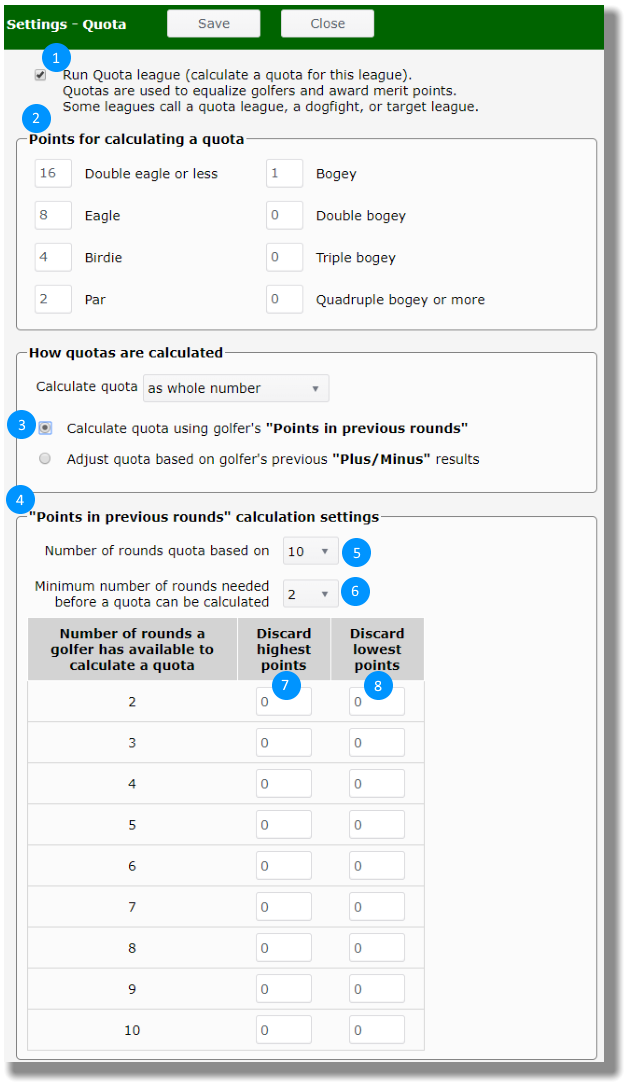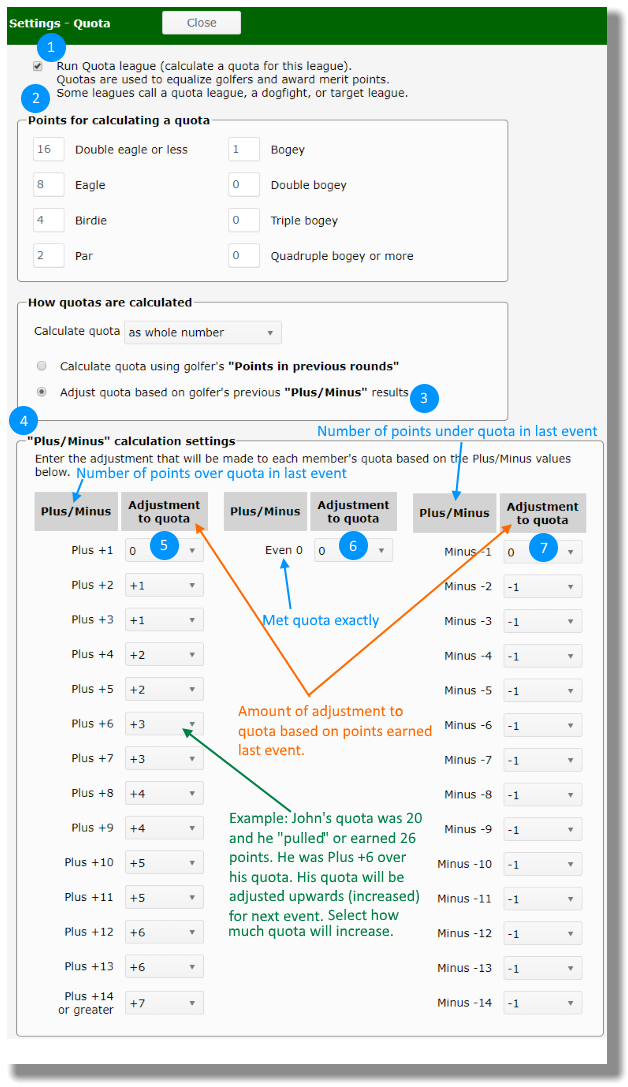Quota Settings

Quota point leagues

Quota point leagues use some form of the Stableford system for awarding points and calculate a quota rather than a handicap. In the Stableford system, a player wins points on each hole based on his score. A player's quota can be based on an average of previous round point totals or adjusted based on the point total in the last round played.

League Manager has two ways to calculate a quota. Select the one which fits what your league does and follow the steps.

Set quota based on points in previous rounds

Open the Main Menu, click Settings, click Quota. The following numbered items correspond to the blue numbered bullets in the image. Note that you can use decimal numbers, if it helps you to break ties for an event.

1. Put a check mark in the box to calculate a quota.
2. Input the points your league awards for eagle, birdie, par, bogie, etc.
3. Click on the option to calculate the quota based on golfer's "Points in previous rounds".
4. Set up calculation settings.
5. Select the most recent number of rounds that the quota will be based on.
6. Select the minimum number of rounds required before a quota will be calculated.
7. For each number of rounds (row of the table), input how many of the highest point totals for a set of rounds will be discarded.
8. For each number of rounds (row of the table), input how many of the lowest point totals for a set of rounds will be discarded.
9. Click the Save button at the top.Set quota based on most recent Plus/Minus result

This method uses your last result to set your quota/target for the next event.

Open the Main Menu, click Settings, click Quota. The following numbered items correspond to the blue numbered bullets in the image.

1. Put a check mark in the box to calculate a quota.
2. Input the points your league awards for eagle, birdie, par, bogie, etc.
3. Click on the option to calculate the quota based on golfer's "most recent Plus/Minus result".
4. Set up calculation settings.
5. For each number of points earned ("pulled") over the quota, select how much quota will go up.
6. For meeting quota exactly, select how much quota will go up or down.
7. For each number of points earned ("pulled") under the quota, select how much quota will go down.
8. Click the Save button at the top.